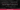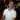# Excel's LEFT(), RIGHT() and MID() String/Text Functions

October 11, 2017

Don’t ask my why, but I love string manipulation. It sounds weird, I know, but I always enjoy finding clever ways to manipulate strings. Whether that would be with regular expressions (a topic to cover later), using Sublime Text to do all sorts of text magic, or using Excel to pick out meaningful data from a seemingly meaningless string. It’s great, I love it. I haven’t covered any Excel text functions yet so I’ll use this as an opportunity to cover the basics of Excel strings and the most common text functions `LEFT()`, `RIGHT()`, and `MID()`. Let’s get started.

## Understanding Strings in Excel

A string in Excel (or Text as they like to call it) can be treated as an array of characters (much like some (most?) programming languages). Normally an array has an index of `0`. Take a look at the following string. Underneath the string is its index.

``````Apple
01234``````

However, Excel’s array indexes start at `1`. So the string should really be considered:

``````Apple
12345``````

Where 1 is the starting index. In Excel’s text functions, you will also see that Excel calls this the `start_num` (short for starting number (i.e. the index)).

So what does this have to do with Excel functions? A lot, actually. While some Excel Text functions return parts of strings, other Excel Text functions return the index of a string (like where you found the letter p in Apple).

I may have an entire post dedicated to strings in Excel, but for now, this amount of understanding should suffice for the functions that follow.## Excel’s LEFT() Text Function

Ever needed to get the first few characters of a string? `LEFT()` is there for you.

Here is the syntax for the `LEFT()` Function:

``LEFT(text, num_of_chars)``
Parameter Meaning
`text` The string you are referencing. Can be a literal string like `Apple` or a reference to a cell like `A1`.
`num_of_chars` Optional. This is short for “number of characters.” If you have text like `Apple` and you ask for `3` characters, you will get `App`. If you don’t specify this parameter, the default is set to `1`.

### Examples of the LEFT() Text Function

#### Get Error Codes

If you had an error log and needed to get the error code, you could use the `LEFT()` Function. If you had this text in `A1:A3`:

``````A1: Error - 012 - Issue on...
A2: Error - 001 - Failed open operation...
A3: Error - 033 - Attempt to read failed...``````

You could get the error codes by getting the first 11 characters:

``````=LEFT(A1, 11) -> Error - 012
=LEFT(A2, 11) -> Error - 001
=LEFT(A3, 11) -> Error - 033``````

#### Get the Log Type

If your log looks like this:

``````A1: Error - 033 - Attempt to read failed...
A2: Warning - Closing before saving...``````

You can use `LEFT()` with `FIND()` where you use `FIND()` to get the first space.

``````=LEFT(A1,FIND(" ",A1)-1) -> Error
=LEFT(A2,FIND(" ",A2)-1) -> Warning``````

The reason for the `-1` in there is because `FIND()` will return the index of where the space is, but if we used that number in the `LEFT()` function, it would include the space, so we subtract `1` in order to remove the trailing space.

## Excel’s RIGHT() Text Function

The `RIGHT()` Function is the opposite of the `LEFT()` Function, where the `RIGHT()` Function will get the last `N` characters from a string.

Here is the syntax for the `RIGHT()` Function:

``RIGHT(text, num_of_chars)``
Parameter Meaning
`text` The string you are referencing. Can be a literal string like `Apple` or a reference to a cell like `A1`.
`num_of_chars` Optional. This is short for “number of characters.” If you have text like `Apple` and you ask for `3` characters, you will get `ple`. If you don’t specify this parameter, the default is set to `1`.

### Example of the RIGHT() Text Function

If you had a list of addresses and they all ended with a zip code, you can use `RIGHT()` to get the zip code:

``````A1: Walt Disney World Resort, Orlando, FL 32830
A2: Statue of Liberty, New York, NY 10004
A3: Yellowstone National Park, <span class="region">WY</span> <span class="postal-code">82190</span>``````

We know that the zip code is 5 characters and we can use that with the RIGHT() Function to our advantage:

``````=RIGHT(A1, 5) -> 32830
=RIGHT(A2, 5) -> 10004
=RIGHT(A3, 5) -> 82190``````

## Excel’s MID() Text Function

If Excel’s `LEFT()` function gets the text on the left side of a string, and Excels `RIGHT()` function gets the text of the right side of a string, then it’s pretty clear that `MID()` should stand for middle (or midway? I’m not actually sure. If you do happen to know what it stands for, please post in the comments below!).

Excel’s `MID()` Function allows you to take a string and start at any position, then take `N` characters from that position and return that portion of the string.

Here is the syntax for the `MID()` Function:

``MID(text, start_num, num_of_chars)``
Parameter Meaning
`text` The string you are referencing. Can be a literal string like `Apple` or a reference to a cell like `A1`.
`start_num` The position of the string where you want to start from. Remember that Excel’s strings start at `1`.
`num_of_chars` This is short for “number of characters” you would like to return.

### Example of the MID() Text Function

#### Get Error Codes From a Log

Let’s go back to our error log for a second:

``````A1: Error - 012 - Issue on...
A2: Error - 001 - Failed open operation...
A3: Error - 033 - Attempt to read failed...``````

If you wanted to get just the error code, you can see that the codes are always a certain length (3 characters) and they always start at the same position (position 9). We can get the error code by using the following formula:

``````=MID(A1,9,3) -> 012
=MID(A2,9,3) -> 001
=MID(A3,9,3) -> 033``````

### Is MID() the Long Version of LEFT() and RIGHT()?

Probably. You can definitely use the `MID()` function just like a `LEFT()` function or a `RIGHT()` function. `LEFT()` just seems like a shorthand way to use `MID()` by automatically specifying the `start_num` to `1`. And `RIGHT()` seems like a shorthand way to specify the `start_num` as the length of the string minus the amount of characters you want.

I imagine that the implementation for LEFT / RIGHT / MID (if it were in VBA) would look something like this:

``````Public Function LEFT(text As String, Optional num_of_chars As Long = 1) As String
LEFT = MID(text, 1, num_of_chars)
End Function

Public Function RIGHT(text As String, Optional num_of_chars As Long = 1) As String
RIGHT = MID(text, Len(text) - num_of_chars, num_of_chars)
End Function

Public Function MID(text As String, start_num As Long, num_of_chars As Long) As String
' Actual Implementation
End Function``````

However, choose whichever option you see fit best. If you always need to start from the beginning of the string, use `LEFT()`, if you always need to start from the end of the string, use `RIGHT()`, otherwise, use `MID()`.

Wow, you read the whole article! You know, people who make it this far are true learners. And clearly, you value learning. Would you like to learn more about Excel? Please consider supporting me by buying me a coffee (it takes a lot of coffee to write these articles!).Written by Joseph who loves teaching about Excel.